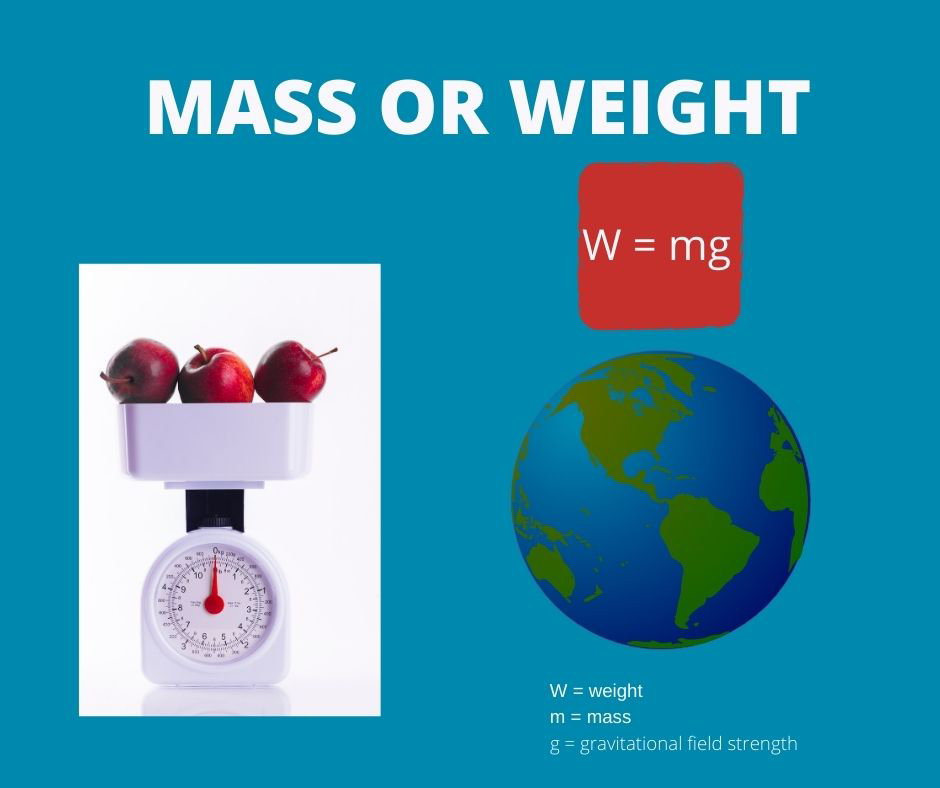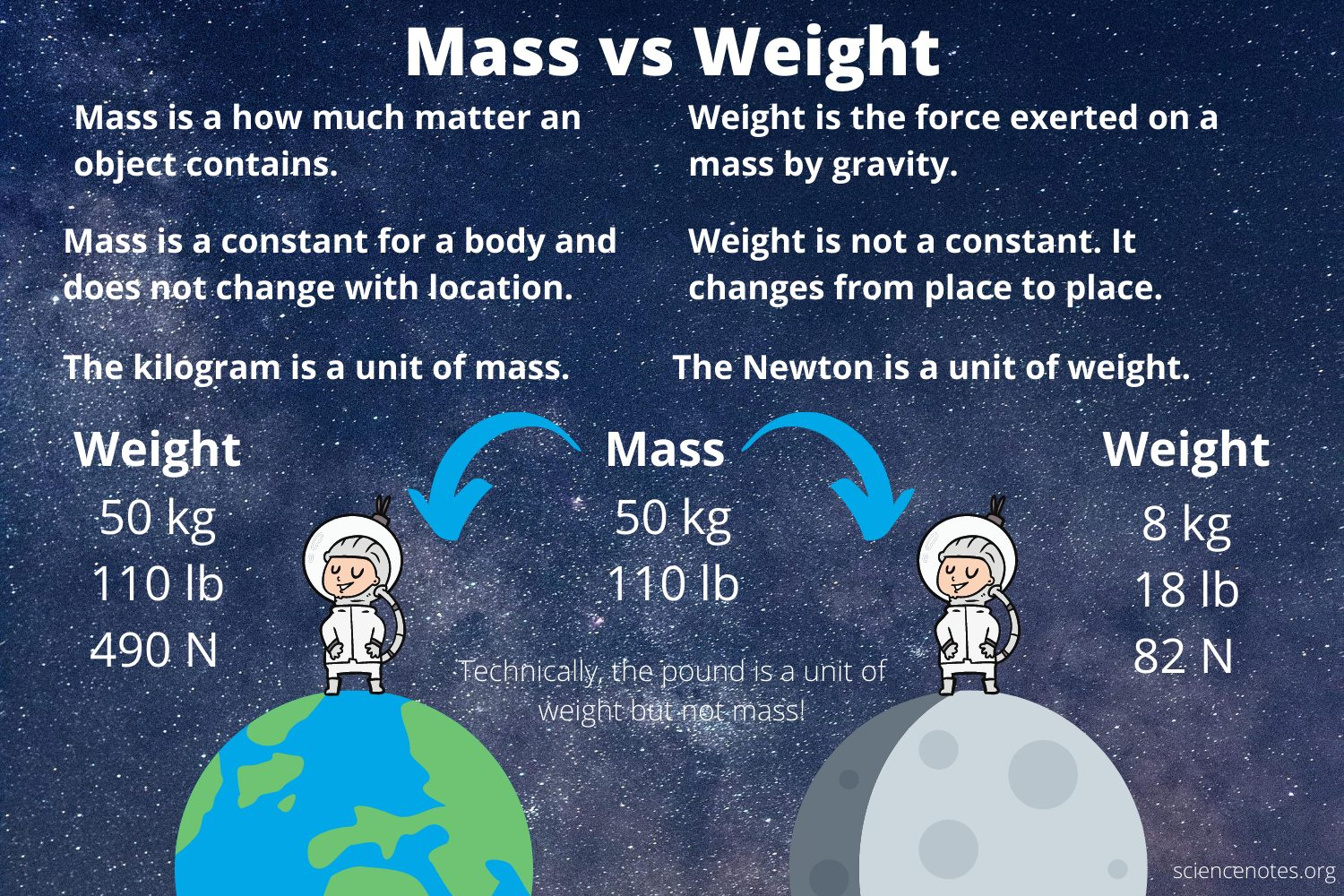HS-PS1-3

# Difference Between Mass and Weight

The mass of a body is the amount of matter contained within it, while the weight of a body is the force acting on it due to acceleration due to gravity. This is the primary difference between mass and weight in chemistry.

## INTRODUCTION

In daily life, the terms mass and weight are often used interchangeably. For students of science, however, it’s crucial to understand the difference. We associate mass and weight with the measurement of how heavy an object is. While this can be a fundamental explanation, there are many details involved. This guide talks about mass and weight and the key differences between them.

### WHAT IS MASS?

Every object in this universe is made of matter, which in turn is made of subatomic particles like electrons, protons, and neutrons. One of the basic properties of matter is that it has a mass. Mass is one of the fundamental quantities in physics, and it is defined as the amount of matter in a particular body.

The international standard unit of mass, or the SI unit, is the Kilogram (Kg). It is crucial to remember that the mass of a body remains constant at all times. However, in certain rare cases like a nuclear reaction, some amount of mass is converted into energy. Therefore, the mass of the substance reduces.

### WHAT IS WEIGHT?

Weight is also one of the fundamental quantities in physics. The definition of weight in chemistry is the amount of gravitational force acting on it. Since weight is a measure of force, the SI unit of weight is Newton.

To find out the weight of a body, we multiple its mass with acceleration due to gravity. Hence, the formula comes out to be, w = mg. Now, in this instant equation, the mass of the body remains constant, but the acceleration due to gravity is variable because it can change from place to place.

When you stand at the North Pole, you experience more weight than a person standing at the equator. The mass of both people here remains the same but acceleration due to gravity changes.

There are two reasons for this:

• Earth is not perfectly spherical, so the person at the equator is farther from Earth's center than the person at the North Pole.
• The acceleration due to gravity is inversely proportional to the square of the distance between two objects.

### WHAT IS THE DIFFERENCE BETWEEN MASS AND WEIGHT IN CHEMISTRY?Source

Let's make a list of properties of both these quantities to understand the basic differences between them.Source

Mass:

• Mass of a body can never be zero.
• Mass only has magnitude, so it is a scalar quantity.
• Mass does not change with location; it remains constant at all times.
• You can use a common balance to measure the mass of a body.
• The SI unit of mass in grams (g) and kilograms (kgs).

Weight:

• The weight of a body can be zero, like in space where there is no force of gravity, so a person experiences weightlessness.
• Weight is a vector quantity because it has magnitude as well as direction.
• Weight of a body changes with location.
• Weight is usually measured using a spring balance. The SI unit of weight is Newton.

## CONCLUSION:

• Mass is one of the basic properties of matter.
• The mass of a body is the measurement of the amount of matter in it.
• The SI unit of mass is the kilogram (kg).
• The mass of a body remains constant at all times.
• Weight is the measurement of the amount of gravitational force acting on a body.
• The SI unit of weight is (N) Newton.
• The weight of a body depends on the location as the acceleration due to gravity is inversely proportional to the square of the distance between two objects.
• There are five basic differences between mass and weight, which are thoroughly listed above.

### FAQs:

1. What is the difference between mass and weight?

The mass of a body is the amount of matter in it, whereas the weight of a body is the measurement of gravitational force acting on it.

2. What are the three differences between mass and weight?

We can write the following points when we state the difference between mass and weight:

• The SI unit of mass is Kilogram, whereas the SI unit of weight is Newton.
• Mass of a body remains constant, whereas weight changes with the place. This is how mass and weight are related.
• Mass only has magnitude, and it is a scalar quantity, whereas weight has magnitude and direction, so it is a vector quantity.

We hope you enjoyed studying this lesson and learned something cool about Mass And Weight! Join our Discord community to get any questions you may have answered and to engage with other students just like you! Don't forget to download our App to experience our fun VR classrooms - we promise it makes studying much more fun! 😎

## SOURCES:

1. Mass and Weight: https://byjus.com/physics/mass-and-weight/.Accessed 28 Feb 2022
2. Mass and Weight: https://www.ck12.org/c/chemistry/mass-and-weight/lesson/SI-Mass-and-Weight-Units-CHEM/.Accessed 28 Feb 2022